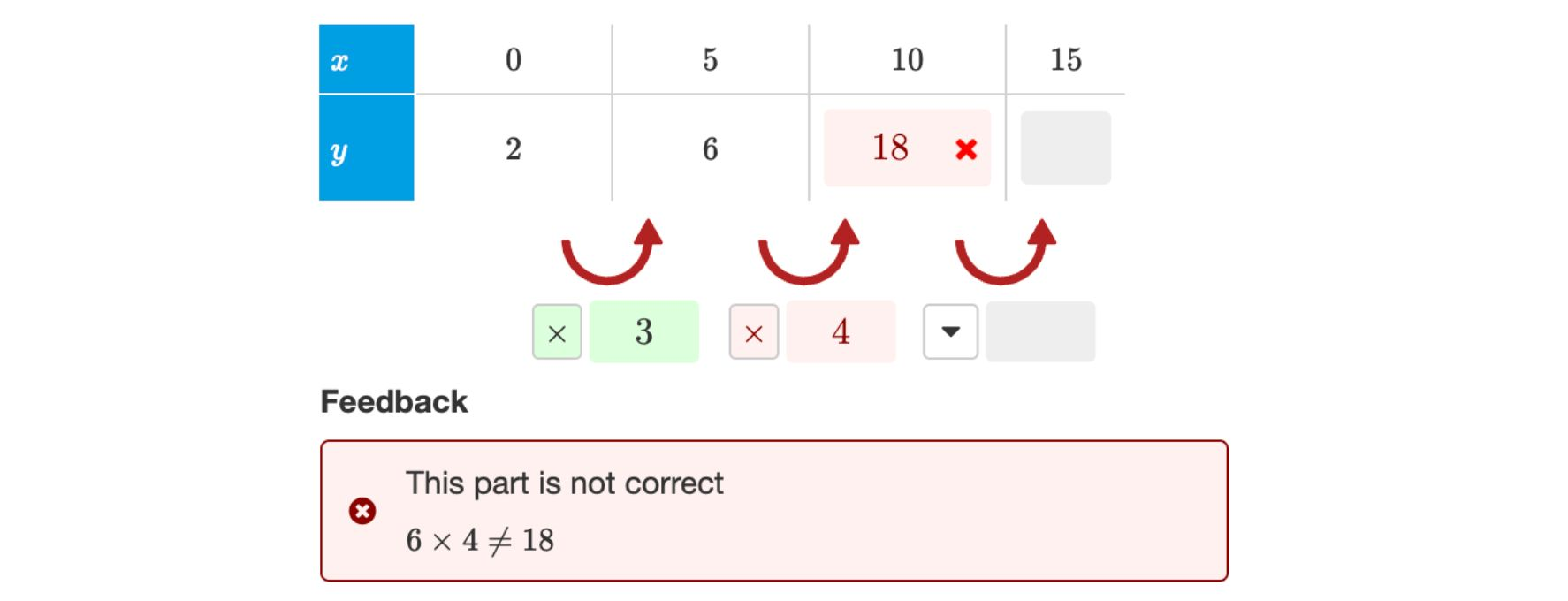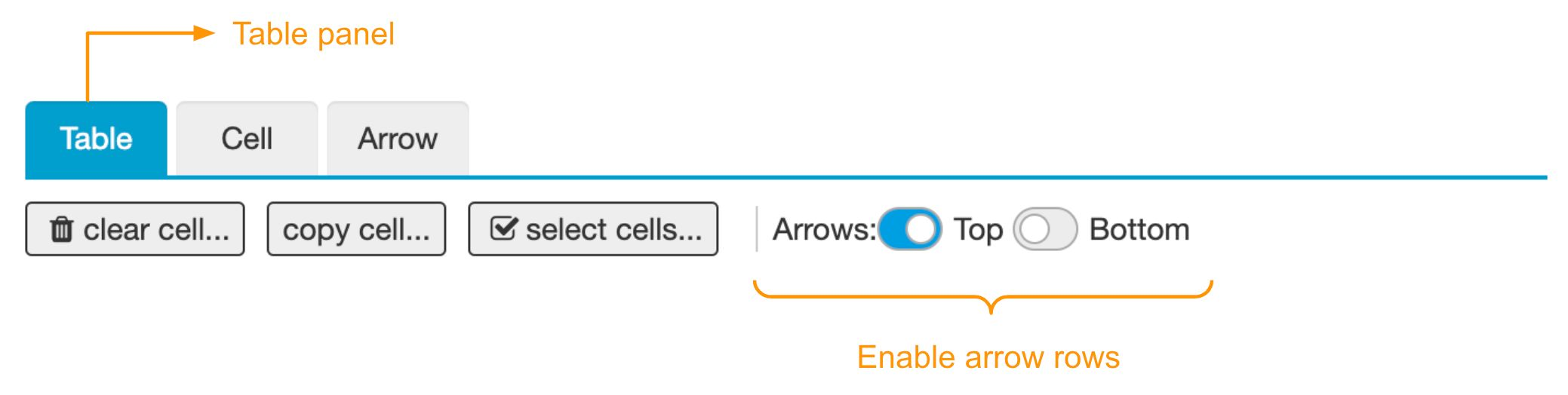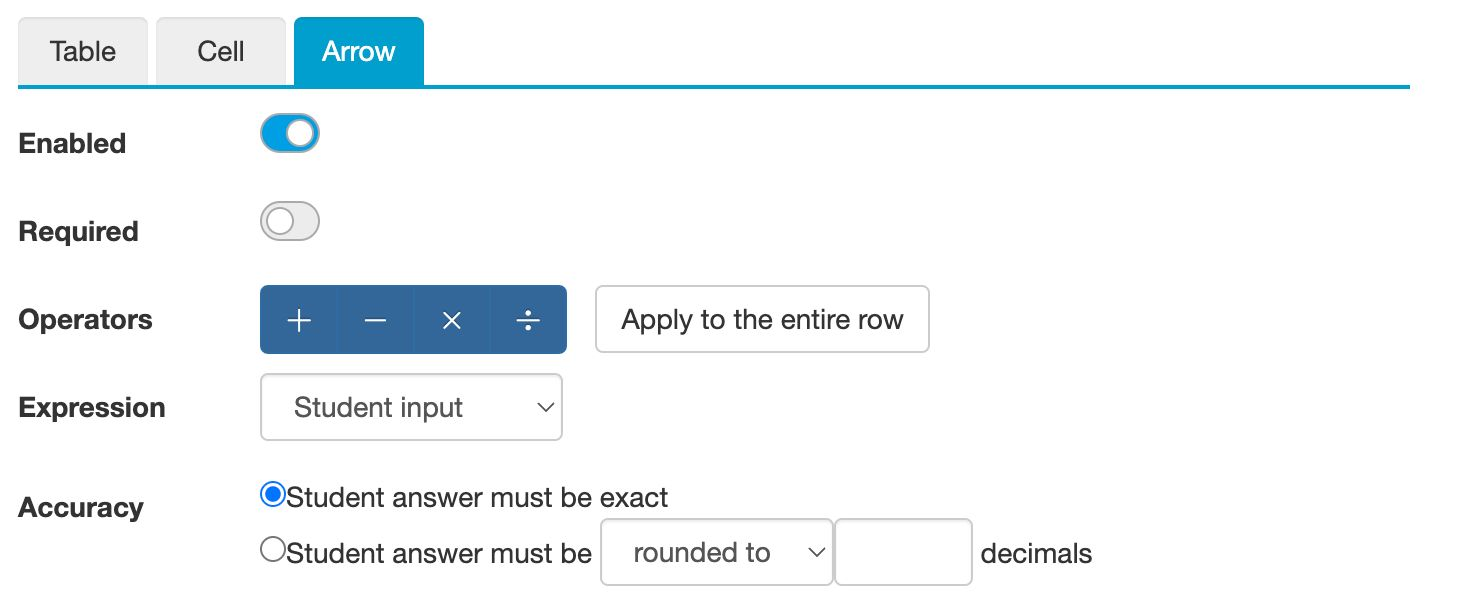# Cell arrows

## Use cases of cell arrows

Math tables sometimes use arrows to emphasize a relation between cells or columns. Examples:

• In Ratio tables, the arrows indicate the multiplication or division between columns. We will discuss ratio tables in more detail later.
• Standard formulas, such as linear, quadratic, exponential, and hyperbolic formulas, use arrows to show the structure of such relations.

Example: A function table of an exponential formula

An arrow is always a relation between the cell before and after. This relation comprises an arithmetic operator and a value, like $+5$ or $\div 2$. Algebrakit will automatically check if the relation is valid. If not, then the operator, the arrow value, or one of the two cells is incorrect. Algebrakit will highlight every cell or arrow that could be incorrect.Figure: Algebrakit checks if each arrow is correct.

## Enabling arrows

You can enable a row of arrows above or below the table using the toggle in the Table panel. You can also disable arrows individually from the Arrow panel.Figure: Enable an arrow row in the table panel.

## Configuring arrows

Click on an arrow to open the Arrow panel.Figure: The Arrow panel.

Use the Enabled toggle to hide the arrow for the student.

If the Required toggle is disabled, students do not have to enter input for the arrow. Use this if you want to use the arrow as an optional aid.

The Operators section configures what arithmetic operators students can choose. If you enable just one operator, then this operator will be visible when the question starts.

In the Expression section, you configure if you want to display an expression or if students should input an expression.

Note

Depending on how you configure the Operators and Expressions sections, you can create arrows for student input or arrows for display. The live example at the top of this page shows some options.

Use the Accuracy section if the input can be a rounded value. Algebrakit will consider this accuracy setting when it evaluates the relation between cells and arrow.

Warning

The accuracy applies to the relation, not the arrow value. For example the relation $3\times 1.667 = 5$ is not accurate to three decimals, because $3\times 1.667 = 5.001$.